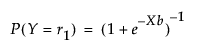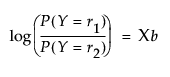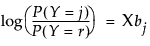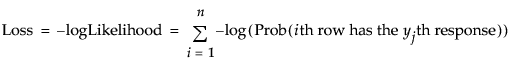Publication date: 08/13/2020

## Logistic Regression Model

Logistic regression fits nominal Y responses to a linear model of X terms. To be more precise, it fits probabilities for the response levels using a logistic function. For two response levels, the function is:where r1 is the first response level

or equivalently:where r1 and r2 are the two responses levels, respectively

Note: When Y is binary and has a nominal modeling type, you can set the Target Level in the Fit Model window to specify the level whose probability you want to model. In this section, the target level is designated as r1.

For r nominal response levels, where r > 2, the model is defined by r 1linear model parameters of the following form:The fitting principal of maximum likelihood means that the βs are chosen to maximize the joint probability attributed by the model to the responses that did occur. This fitting principal is equivalent to minimizing the negative log-likelihood (–LogLikelihood):